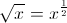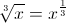## Example Questions

← Previous 1 3

### Example Question #1 : How To Find Patterns In Exponents

If ax·a4 = a12 and (by)3 = b15, what is the value of x - y?

-4

-9

3

6

-2

3

Explanation:

Multiplying like bases means add the exponents, so x+4 = 12, or x = 8.

Raising a power to a power means multiply the exponents, so 3y = 15, or y = 5.

x - y = 8 - 5 = 3.

### Example Question #2 : How To Find Patterns In Exponents

If p and q are positive integrers and 27= 9q, then what is the value of q in terms of p?

3p

p

(3/2)p

(2/3)p

2p

(3/2)p

Explanation:

The first step is to express both sides of the equation with equal bases, in this case 3. The equation becomes 33p = 32q. So then 3p = 2q, and q = (3/2)p is our answer.

### Example Question #3 : How To Find Patterns In Exponents

Simplify 272/3.

729

125

9

3

27

9

Explanation:

272/3 is 27 squared and cube-rooted. We want to pick the easier operation first. Here that is the cube root. To see that, try both operations.

272/3 = (272)1/3 = 7291/3 OR

272/3 = (271/3)2 = 32

Obviously 32 is much easier. Either 32 or 7291/3 will give us the correct answer of 9, but with 32 it is readily apparent.

### Example Question #4 : How To Find Patterns In Exponents

Ifandare integers and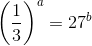what is the value of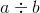?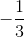Explanation:

To solve this problem, we will have to take the log of both sides to bring down our exponents. By doing this, we will get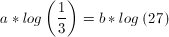.

To solve for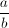we will have to divide both sides of our equation by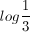to get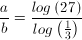.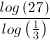will give you the answer of –3.

### Example Question #5 : How To Find Patterns In Exponents

If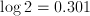and, then what is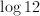?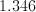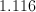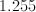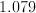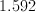Explanation:

We use two properties of logarithms: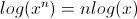So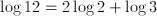### Example Question #6 : How To Find Patterns In Exponents

Evaluate: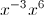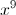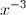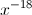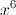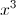Explanation: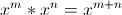, here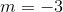and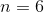, hence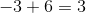.

### Example Question #7 : How To Find Patterns In Exponents

Solve for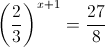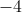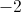None of the aboveExplanation:=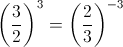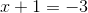which means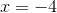### Example Question #8 : How To Find Patterns In Exponents

Which of the following statements is the same as: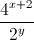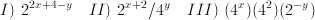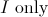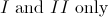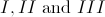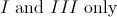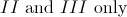Explanation:

Remember the laws of exponents. In particular, when the base is nonzero: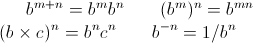An effective way to compare these statements, is to convert them all into exponents with base 2. The original statement becomes: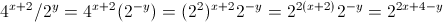This is identical to statement I. Now consider statement II: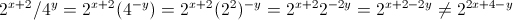Therefore, statement II is not identical to the original statement. Finally, consider statement III: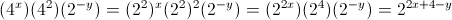which is also identical to the original statement. As a result, only I and III are the same as the original statement.

### Example Question #9 : How To Find Patterns In Exponents

Write in exponential form: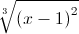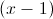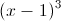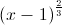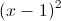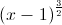Explanation:

Using properties of radicals e.g.,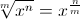we get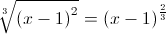### Example Question #10 : How To Find Patterns In Exponents

Write in exponential form: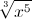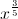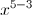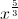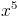Explanation: